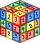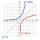# Geometric progression

In geometric progression, a1 = 7, q = 5. Find the condition for n to sum first n members is: sn≤217.

Result

n =  3

#### Solution:Leave us a comment of example and its solution (i.e. if it is still somewhat unclear...):Be the first to comment!## Next similar examples:

1. Six termsFind the first six terms of the sequence a1 = -3, an = 2 * an-1
2. Geometric sequence 4It is given geometric sequence a3 = 7 and a12 = 3. Calculate s23 (= sum of the first 23 members of the sequence).
3. GP - 8 itemsDetermine the first eight members of a geometric progression if a9=512, q=2
4. Five membersWrite first 5 members geometric sequence and determine whether it is increasing or decreasing: a1 = 3 q = -2
5. GP membersThe geometric sequence has 10 members. The last two members are 2 and -1. Which member is -1/16?
6. SequenceCalculate what member of the sequence specified by ? has value 86.
7. Investment1000\$ is invested at 10% compound interest. What factor is the capital multiplied by each year? How much will be there after n=12 years?A radioactive material loses 10% of its mass each year. What proportion will be left there after n=6 years?
9. Tenth memberCalculate the tenth member of geometric sequence when given: a1=1/2 and q=2
10. QuotientDetermine the quotient and the second member of the geometric progression where a3=10, a1+a2=-1,6 a1-a2=2,4.
11. Geometric progression 2There is geometric sequence with a1=5.7 and quotient q=-2.5. Calculate a17.
12. The city 3The city has 22,000 residents. How long it is expected to have 25,000 residents if the average annual population growth is 1.4%?
13. Exponential equationIn the set R solve the equation: ?
14. Logif ?, what is b?
15. LogarithmDetermine the number whose decimal logarithm is -3.8.
16. CoordinateDetermine missing coordinate of the point M [x, 120] of the graph of the function f bv rule: y = 5x
17. Theorem proveWe want to prove the sentence: If the natural number n is divisible by six, then n is divisible by three. From what assumption we started?